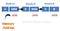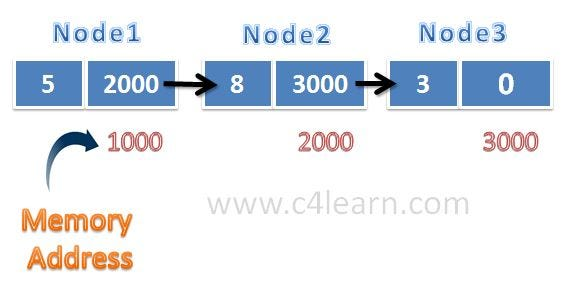# .css-4zleql{display:block;}Vinit Raut## Introduction

Data Structures are one of the most important parts of programming. Real-world data is often unstructured and raw. In order to perform operations on data, we need to organize data in an efficient way. This is where data structures come into play. Having a good grasp of data structures help us write efficient code to develop faster, efficient, and scalable applications. They organize data in such a way that we can easily perform various operations like insert, update, delete, traverse, etc.

There are many data structures for different use cases, one of the most popular and hot topics for interviews are Linked Lists. Today, we will take a deep dive into linked lists. By the end, you’ll know what a linked list is, how to create a linked list, and perform various operations like insertion, and deletion.

In arrays, we need to specify the size before using it. In a practical scenario, we can’t predict what would be the size that is required to store all the data in an array. If we specify a size larger than what is required, we may end up wasting the non-used memory. If we specify a size smaller than what is required, we may not be able to fit all the data in an array. To overcome this, we have another data structure called the Linked list. A linked list is a non-primitive data structure where nodes are connected to each other that are stored in a non-contiguous memory location.

## Basic Structure of Linked List

A Linked list is a non-primitive linear data structure. Unlike arrays, they aren’t stored in contiguous memory locations. They are stored in a random memory location where the first node is connected to the second node, the first node has the address of the subsequent node, and so on. The last node has null in the next field that depicts its the end of the linked list.The basic structure of a linked list

Let’s create our first node since there is no primitive data type to store data and address together, we create a class Node to store data and address together.

`````` public class Node<T> {
T data;
Node<T> next;

Node(T data) {
this.data = data;
next = null;
}
}
``````

Linked List can contain any primitive or non-primitive data. Hence, we have defined it generic. We define a constructor where we will pass the data to create a node. By default, next would be null. We can later update the next field to link it to another node.

## Taking input and creating a node

After specifying a Node class, we can simply create a node (integer) with the following syntax:

``````  Node<Integer> node1 = new Node<Integer>(10);
``````

We have created our first node with data 10 but creating multiple nodes using this approach is not efficient. We can create a takeInput function for this purpose.

``````/* Function to take input of a linked list */

public static Node<Integer> takeInput() {
Scanner s = new Scanner(System.in);
Node<Integer> head = null, tail = null;
int data = s.nextInt();
while (data != -1) {
Node<Integer> newNode = new Node<Integer>(data);
tail = newNode;
} else {
tail.next = newNode;
tail = newNode;
}
data = s.nextInt();
}
s.close();
}
/* Time Complexity: O(N) */
``````

We can print all the elements from a linked list by traversing each node and printing it. Let’s create a printList function for this operation:

``````/* Function to print a linked list */
public static void printList(Node<Integer> head) {
return;
}
}
}

/* Time Complexity: O(N) */
``````

## Insertion in a Linked list

We can insert a new node at the beginning, end, or any specified position. The code for the insertion function is the following:

``````/* Function to insert node in a linked list */
public static Node<Integer> insert(Node<Integer>, int data, int pos) {
Node<Integer> newNode = new Node<Integer>(data);
if (pos == 0) {
return newNode;
}
int i = 0;
while (temp != null && i < pos - 1) {
temp = temp.next;
i++;
}
newNode.next = temp.next;
temp.next = newNode;
}

/* Time Complexity: O(N) */
``````

## Deletion in a Linked list

Like insertion, we can also delete from the beginning, end, or any specified position. The code for the deletion function is the following:

``````/* Function to remove node from a linked list */
public static Node<Integer> delete(Node<Integer> data, int pos) {
}
int i = 0;
if (pos == 0) {
}
while (temp != null && i < pos - 1) {
temp = temp.next;
i++;
}
if (temp != null && temp.next != null) {
temp.next = temp.next.next;
}
}

/* Time Complexity: O(N) */
``````

1. Singly Linked List — It contains a data field that contains data and a next field that contains the address of the next node.

2. Doubly Linked List — It has 3 fields, the data field contains data, a prev field that has the address of the previous node, and a next field that has the address of the next node.

3. Circular Linked List — In a circular linked list, the last node has the address of the first node forming a circular chain, hence the name.

Every data structure has its own advantages and disadvantages depending upon its use case. It’s important to know where linked lists do well and where not before using them.

Pros

1. A linked list is best suited for the size of the structure that is constantly changing.
2. The only required amount of nodes is created. Hence, there is no need to specify size at the beginning, this overcomes the limitation of the static arrays.

Cons

1. Extra memory is needed to store the address of the contiguous node.
2. No element can be accessed randomly; each node is traversed sequentially.
3. In a singly linked list, traversal from end to start is not possible.

## Conclusion

• Linked lists are a non-primitive linear data structure where each node contains data and address to the next node.
• No need to specify the size at the beginning like arrays, best suited for dynamic nature where size is constantly changing.
• We can perform various operations like insert, update, delete, traverse, etc easily.
• We can implement other data structures like stacks, queues, etc with the help of linked lists.
• If you like learning by watching videos, here’s a good playlist for Data Structures and Algorithms by mycodeschool

I hope you got a fair understanding of what a linked list is and how can we perform basic operations on them. If you have any feedback or suggestions, feel free to reach out to me on Twitter or LinkedIn

#data-structures#java#programming-ciovqvfcb008mb253jrczo9ye